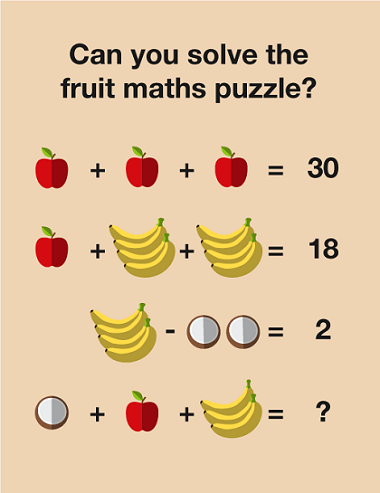Everyday maths 2 (Wales)

Start this free course now. Just create an account and sign in. Enrol and complete the course for a free statement of participation or digital badge if available.

# 1 Four operations

You will already be using the four operations in your daily life (whether you realise it or not). Everyday life requires you to carry out maths all the time; checking you’ve been given the correct change, working out how many packs of cakes you need for the children’s birthday party and splitting the bill in a restaurant are all examples that come to mind.Figure 1 Fruit maths puzzle

The four operations are addition, subtraction, multiplication and division. You need to understand what each operation does and when to use it and at level 2, you will often be required to use more than one operation to answer a question.

• This operation is used when you want to find the total, or sum, of two or more amounts.

• Subtraction (−)

• This operation is used when you want to find the difference between two amounts or how much of something you have left after a quantity is used – for example, if you want to find the change owed after spending an amount of money.

• Multiplication (×)

• This operation is used to calculate multiple amounts of the same number. For example, if you wanted to know the cost of 16 plants costing £4.75 each you would multiply £4.75 by 16:

£4.75 × 16 = £76

• Division (÷)

• Division is used when sharing or grouping items. For example, if a group of 6 friends won £765 on the lottery and you wanted to know how much each person would get you would divide £765 by 6:

£765 ÷ 6 = £127.50

If you are doing this course to prepare you for Essential Skills Wales Application of Number, remember the exam papers do not allow the use of a calculator so please attempt the calculations in this session by hand.

## Activity 1: Operation choice

Each of the four questions below uses one of the four operations. Match the operation to the question.

Using the following two lists, match each numbered item with the correct letter.

1.2.3.4.• a.Fourteen members of the same family go on holiday together. They each pay £155. What is the total cost of the holiday?

• b.You go to the local café and buy a coffee for £2.35, a tea for £1.40 and a croissant for £1.85. How much do you spend?

• c.You need to save £306 for a holiday. You have 18 months to save up that much money. How much do you need to save per month?

• d.You make an insurance claim worth £18 950. The insurance company pays you £12 648. What is the difference between what you claimed and what you actually received?

• 1 = c
• 2 = a
• 3 = d
• 4 = b

Try these questions to make sure that you are confident using each of the four operations.

## Activity 2: Calculations with whole numbers

1. 1245 + 654

2. 187 + 65 401

3. 1060 − 264

4. 2000 − 173

5. 543 × 19

6. 1732 × 46

7. 1312 ÷ 8

8. 1044 ÷ 12

9. Stuart is saving £225 per month for a new car. How much would he have saved in:

• a.1 year

• b.2 years

10. A concert venue has 2190 seats arranged into 15 sections. How many seats are in each section?

11. A college sells 325 tickets to the charity ball at £12 each. How much money do they make from ticket sales?

1. 1245 + 654 = 1899

2. 187 + 65 401 = 65 588

3. 1060 − 264 = 796

4. 2000 − 173 = 1827

5. 543 × 19 = 10 317

6. 1732 × 46 = 79 672

7. 1312 ÷ 8 = 164

8. 1044 ÷ 12 = 87

• a.Stuart is saving £225 per month for 1 year or 12 months so the calculation is 225 × 12 = £2700.

• b.Stuart saves £2700 in 1 year so to work out the amount saved in 2 years the calculation is 2 × £2700 = £5400. Alternatively, you could add £2700 + £2700 = £5400.

9. To calculate this you divide the number of seats by the number of sections.

So, 2190 ÷ 15 = 146. So there are 146 seats in each section.

10. To calculate this you multiply the number of tickets by the cost per ticket.

So, 325 × 12 = 3900. So the college will make £3900 from ticket sales.#### Find out more

*Eligibility rules apply for financial support.